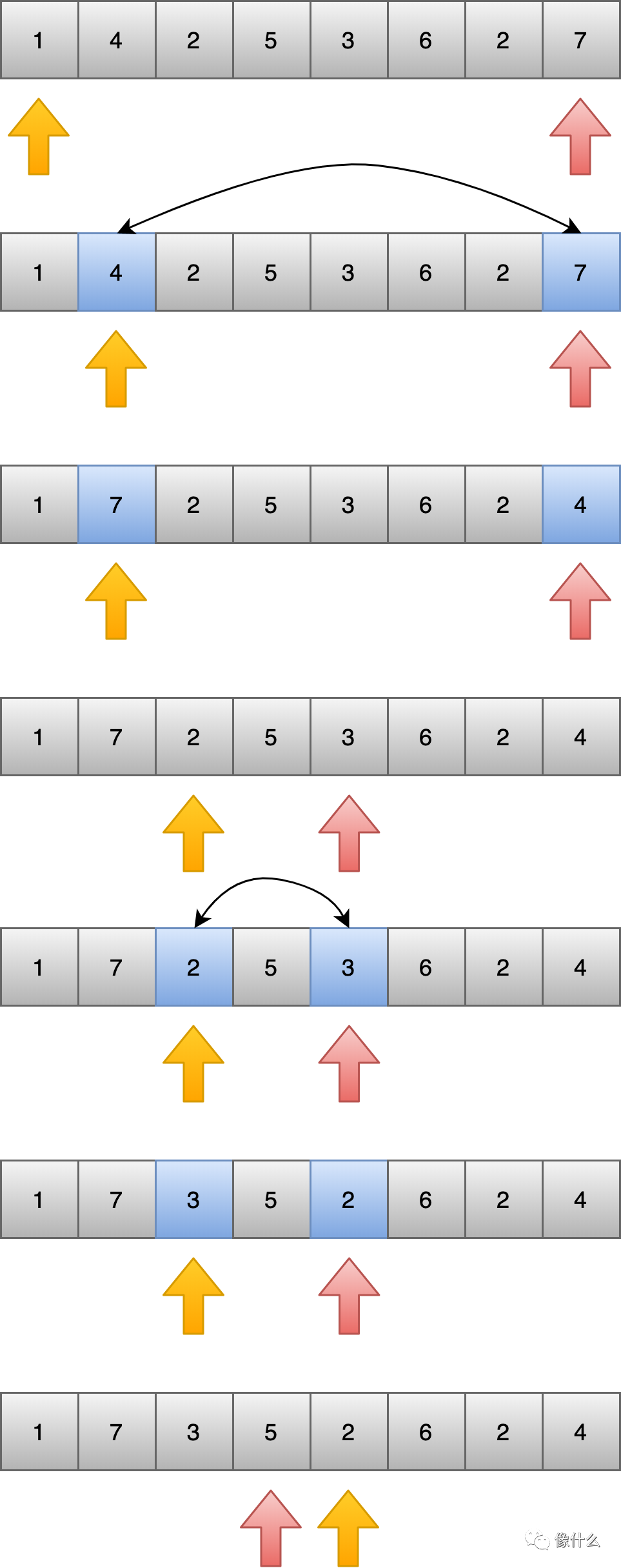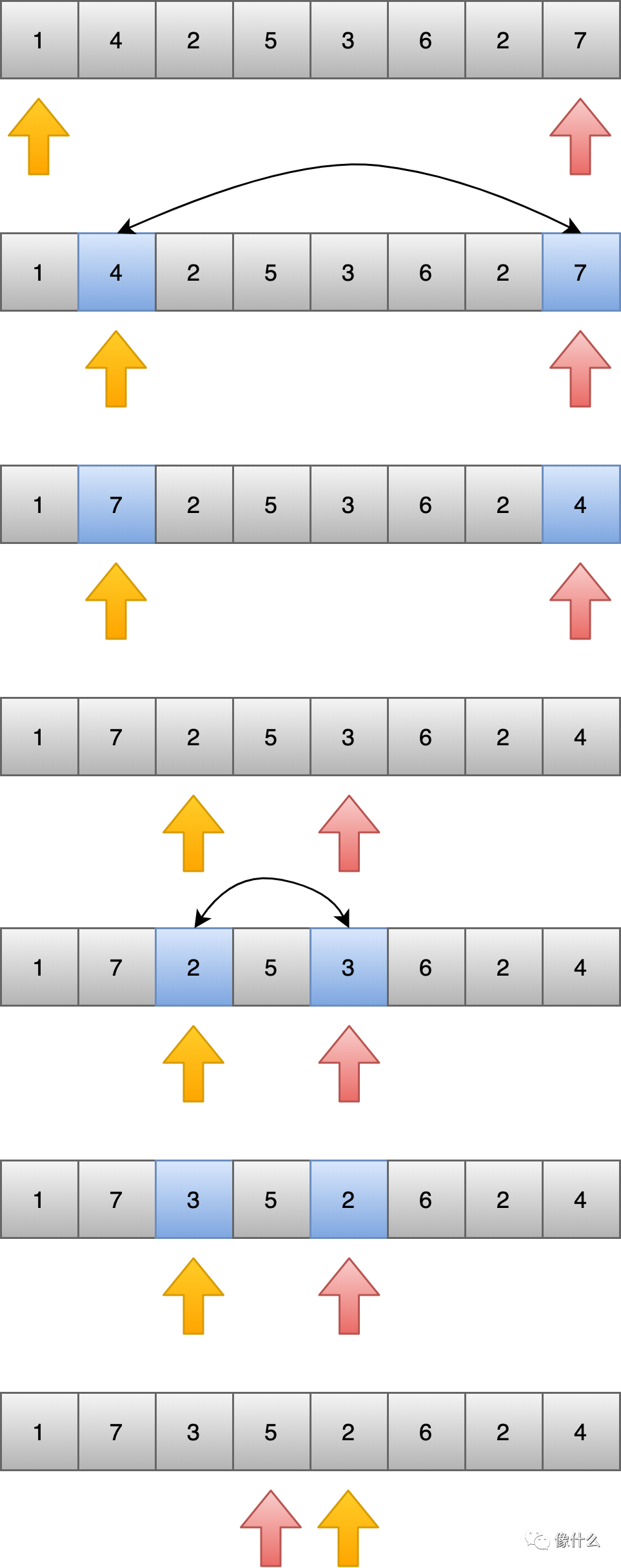# 两边夹的应用二public void reorderByOddEven(int[] ints) {    if (ints == null || ints.length < 2) {        return;    }    for (int left = 0, right = ints.length - 1; left < right; ) {        while (left < ints.length && (ints[left] & 1) == 1) {            left++;        }        while (right >= 0 && (ints[right] & 1) == 0) {            right--;        }        if (left < right) {            swap(ints, left, right);        }    }}privat void swap(int[] ints, int left, int right) {    int temp = ints[right];    ints[right] = ints[left];    ints[left] = temp;}

while ((ints[left] & 1) == 1) {    left++;    if (left >= ints.length) {        break;    }}while ((ints[right] & 1) == 0) {    right--;    if (right < 0) {        break;    }}

@FunctionalInterfacepublic interface ReorderCondition {    boolean check(int num);}

private void reorderByCondition(int[] ints, ReorderCondition reorderCondition) {    if (ints == null || ints.length < 2) {        return;    }    for (int left = 0, right = ints.length - 1; left < right; ) {        while (left < ints.length && reorderCondition.check(ints[left])) {            left++;        }        while (right >= 0 && !reorderCondition.check(ints[right])) {            right--;        }        if (left < right) {            swap(ints, left, right);        }    }}

reorderByCondition(ints, num -> {    return (num & 1) == 1;});//或者为了条件可以重用，先定义再引用ReorderCondition oddEven = num -> {    return (num & 1) == 1;};reorderByCondition(ints, oddEven);

reorderByCondition(ints, num -> {    return (num % 3) == 0;});## 评论